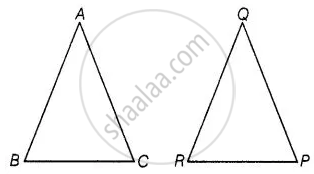# If in two triangles ABC and PQR, ABQR=BCPR=CAPQ, then ______. - Mathematics

MCQ
Fill in the Blanks

If in two triangles ABC and PQR, (AB)/(QR) = (BC)/(PR) = (CA)/(PQ), then ______.

• ΔPQR ~ ΔCAB

• ΔPQR ~ ΔABC

• ΔCBA ~ ΔPQR

• ΔBCA ~ ΔPQR

#### Solution

If in two triangles ABC and PQR, (AB)/(QR) = (BC)/(PR) = (CA)/(PQ), then ΔPQR ~ ΔCAB.

Explanation:

Given, in two ΔABC and ΔPQR,

(AB)/(QR) = (BC)/(PR) = (CA)/(PQ)

Which shows that sides of one triangle are proportional to the side of the other triangle, then their corresponding angles are also equal, so by SSS similarity, triangles are similar.

i.e., ΔCAB ∼ ΔPQRConcept: Similarity of Triangles
Is there an error in this question or solution?

#### APPEARS IN

NCERT Mathematics Exemplar Class 10
Chapter 6 Triangles
Exercise 6.1 | Q 4 | Page 61
Share